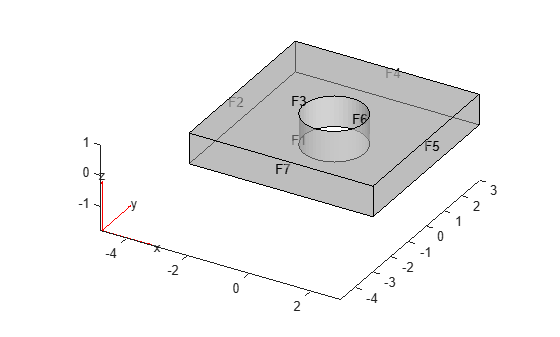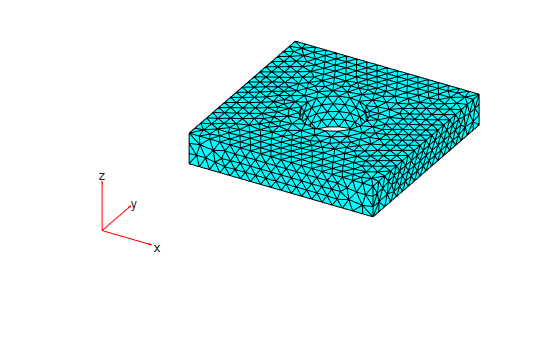# Geometry from `alphaShape`

Create a 3-D geometry using the MATLAB® `alphaShape` function. First, create an `alphaShape` object of a block with a cylindrical hole. Then create a geometry from the `alphaShape` boundary.

Create a 2-D mesh grid.

```[xg,yg] = meshgrid(-3:0.25:3); xg = xg(:); yg = yg(:);```

Create a unit disk. Remove all the mesh grid points that fall inside the unit disk, and include the unit disk points.

```t = (pi/24:pi/24:2*pi)'; x = cos(t); y = sin(t); circShp = alphaShape(x,y,2); in = inShape(circShp,xg,yg); xg = [xg(~in); cos(t)]; yg = [yg(~in); sin(t)];```

Create 3-D copies of the remaining mesh grid points, with the z-coordinates ranging from 0 through 1. Combine the points into an `alphaShape` object.

```zg = ones(numel(xg),1); xg = repmat(xg,5,1); yg = repmat(yg,5,1); zg = zg*(0:.25:1); zg = zg(:); shp = alphaShape(xg,yg,zg);```

Generate a surface mesh of the `alphaShape` object.

`[elements,nodes] = boundaryFacets(shp);`

Create an `fegeometry` object from the surface mesh.

`gm = fegeometry(nodes,elements)`
```gm = fegeometry with properties: NumFaces: 7 NumEdges: 14 NumVertices: 10 NumCells: 1 Vertices: [10x3 double] Mesh: [] ```

For a 3-D geometry created from the surface mesh, the `Mesh` property remains empty. To use the geometry in an analysis, generate a mesh.

```gm = generateMesh(gm); gm.Mesh```
```ans = FEMesh with properties: Nodes: [3x10812 double] Elements: [10x6569 double] MaxElementSize: 0.3418 MinElementSize: 0.1709 MeshGradation: 1.5000 GeometricOrder: 'quadratic' ```

Plot the geometry with the face labels.

`pdegplot(gm,FaceLabels="on",FaceAlpha=0.5);`Plot the mesh.

`pdemesh(gm);`